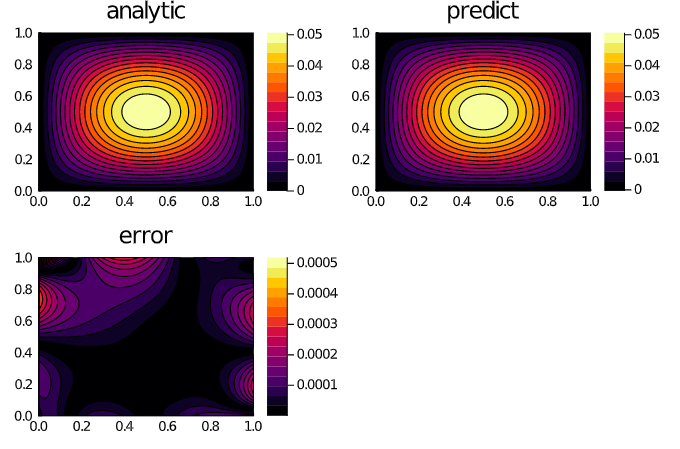## NeuralPDE.jl

Physics-Informed Neural Networks (PINN) and Deep BSDE Solvers of Differential Equations for Scientific Machine Learning (SciML) accelerated simulation
Author SciML
Popularity
327 Stars
Updated Last
1 Year Ago
Started In
March 2017

# NeuralPDE

NeuralPDE.jl is a solver package which consists of neural network solvers for partial differential equations using scientific machine learning (SciML) techniques such as physics-informed neural networks (PINNs) and deep BSDE solvers. This package utilizes deep neural networks and neural stochastic differential equations to solve high-dimensional PDEs at a greatly reduced cost and greatly increased generality compared with classical methods.

## Installation

Assuming that you already have Julia correctly installed, it suffices to install NeuralPDE.jl in the standard way, that is, by typing `] add NeuralPDE`. Note: to exit the Pkg REPL-mode, just press Backspace or Ctrl + C.

## Tutorials and Documentation

For information on using the package, see the stable documentation. Use the in-development documentation for the version of the documentation, which contains the unreleased features.

## Features

• Physics-Informed Neural Networks for automated PDE solving
• Forward-Backwards Stochastic Differential Equation (FBSDE) methods for parabolic PDEs
• Deep-learning-based solvers for optimal stopping time and Kolmogorov backwards equations

## Example: Solving 2D Poisson Equation via Physics-Informed Neural Networks

```using NeuralPDE, Flux, ModelingToolkit, GalacticOptim, DiffEqFlux

@parameters x y
@variables u(..)
Dxx = Differential(x)^2
Dyy = Differential(y)^2

# 2D PDE
eq  = Dxx(u(x,y)) + Dyy(u(x,y)) ~ -sin(pi*x)*sin(pi*y)

# Boundary conditions
bcs = [u(0,y) ~ 0.f0, u(1,y) ~ -sin(pi*1)*sin(pi*y),
u(x,0) ~ 0.f0, u(x,1) ~ -sin(pi*x)*sin(pi*1)]
# Space and time domains
domains = [x ∈ IntervalDomain(0.0,1.0),
y ∈ IntervalDomain(0.0,1.0)]
# Discretization
dx = 0.1

# Neural network
dim = 2 # number of dimensions
chain = FastChain(FastDense(dim,16,Flux.σ),FastDense(16,16,Flux.σ),FastDense(16,1))

pde_system = PDESystem(eq,bcs,domains,[x,y],[u])
prob = discretize(pde_system,discretization)

cb = function (p,l)
println("Current loss is: \$l")
return false
end

res = GalacticOptim.solve(prob, ADAM(0.1); cb = cb, maxiters=4000)
prob = remake(prob,u0=res.minimizer)
res = GalacticOptim.solve(prob, ADAM(0.01); cb = cb, maxiters=2000)
phi = discretization.phi```

And some analysis:

```xs,ys = [domain.domain.lower:dx/10:domain.domain.upper for domain in domains]
analytic_sol_func(x,y) = (sin(pi*x)*sin(pi*y))/(2pi^2)

u_predict = reshape([first(phi([x,y],res.minimizer)) for x in xs for y in ys],(length(xs),length(ys)))
u_real = reshape([analytic_sol_func(x,y) for x in xs for y in ys], (length(xs),length(ys)))
diff_u = abs.(u_predict .- u_real)

using Plots
p1 = plot(xs, ys, u_real, linetype=:contourf,title = "analytic");
p2 = plot(xs, ys, u_predict, linetype=:contourf,title = "predict");
p3 = plot(xs, ys, diff_u,linetype=:contourf,title = "error");
plot(p1,p2,p3)```## Example: Solving a 100-Dimensional Hamilton-Jacobi-Bellman Equation

```using NeuralPDE
using Flux
using DifferentialEquations
using LinearAlgebra
d = 100 # number of dimensions
X0 = fill(0.0f0, d) # initial value of stochastic control process
tspan = (0.0f0, 1.0f0)
λ = 1.0f0

g(X) = log(0.5f0 + 0.5f0 * sum(X.^2))
f(X,u,σᵀ∇u,p,t) = -λ * sum(σᵀ∇u.^2)
μ_f(X,p,t) = zero(X)  # Vector d x 1 λ
σ_f(X,p,t) = Diagonal(sqrt(2.0f0) * ones(Float32, d)) # Matrix d x d
prob = TerminalPDEProblem(g, f, μ_f, σ_f, X0, tspan)
hls = 10 + d # hidden layer size
# sub-neural network approximating solutions at the desired point
u0 = Flux.Chain(Dense(d, hls, relu),
Dense(hls, hls, relu),
Dense(hls, 1))
# sub-neural network approximating the spatial gradients at time point
σᵀ∇u = Flux.Chain(Dense(d + 1, hls, relu),
Dense(hls, hls, relu),
Dense(hls, hls, relu),
Dense(hls, d))
pdealg = NNPDENS(u0, σᵀ∇u, opt=opt)
@time ans = solve(prob, pdealg, verbose=true, maxiters=100, trajectories=100,
alg=EM(), dt=1.2, pabstol=1f-2)```

### Required Packages

View all packages

### Used By Packages

No packages found.